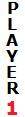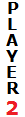# How to Calculate Gambler's Ruin

Probability of Gambler's RuinProbability of Winning One Round =
Number of Chips =Probability of Winning One Round =
Number of Chips =

In probability theory, Gambler's Ruin is the likelihood that one player will run out of money after playing a series of betting games. In a two-player game, each player's probability of ruin depends on several variables: the number of chips each player has, and each player's probability of winning one game. The equations for the probabilities of each player's ruin are

R1 = [1 - (p/q)K]/[1 - (p/q)K+N]
R2 = [1 - (q/p)N]/[1 - (q/p)N+K],

where p is the probability that Player 1 wins a single game and q = 1-p is the probability that Player 2 wins a single game. N is the number of chips that Player 1 possesses and K is the number of chips that Player 2 possesses. The rules of the game are that if one player loses a round, he gives the other player one of his chips.

In case p = q = 0.5, the probabilities of ruin are given by the equations

R1 = K/(K+N)
R2 = N/(N+K)

Notice that in both cases, R1 + R2 = 1. If you want to find the probability that a player eventually wins all of the chips, just switch R1 and R2. That is, the probability that Player 1 wins all the chips eventually is equivalent to the probability of Player 2's ruin.

Example 1

Anne and Carol play a game by rolling two dice. If the sum of the dice is 8 or more, Anne wins. If the sum of the dice is 7 or less, Carol wins. Furthermore, Anne starts with 23 chips and Carol starts with 9 chips. Let's use this information to find the probabilities that Anne or Carol lose all of their chips.

If we call Anne Player 1, then p = 5/12 and N = 23. (To calculate p, notice that there are 36 ways to roll two dice and 15 of those outcomes have a sum of 8 or more. Thus, p = 15/36 or 5/12.).

If we call Carol Player 2, then q = 7/12 and K = 9. Therefore, we have

R1 = [1 - (5/7)9]/[1 - (5/7)32] = 0.95162
R2 = [1 - (7/5)23]/[1 - (7/5)32] = 0.04838

So the chance that Anne eventually runs out of chips is 95.162%. Equivalently, the probability that Carol wins all of the chips in the end is 95.162%. Even though Anne has more chips than Carol, it doesn't mitigate the fact that the game is heavily weighted in Carol's favor.

Example 2

Bob and Fred flip a penny, and Bob wins if the penny lands on heads. Assume that Bob has 7 chips and Fred has 13. If we call Bob Player 1, then p = 0.5 and N = 7. If we call Fred Player 2, then q = 0.5 and K = 13. We use the second set of formulas to compute the probability of ruin:

R1 = 13/20 = 0.65
R2 = 7/20 = 0.35

Thus, Bob's chances of losing all of his chips is 65%.